1602745200

# Exploring The Brute Force K-Nearest Neighbors Algorithm

Did you find any difference between the two graphs?

Both show the accuracy of a classification problem for K values between 1 to 10.

Both of the graphs use the KNN classifier model with ‘Brute-force’ algorithm and ‘Euclidean’ distance metric on same dataset. Then why is there a difference in the accuracy between the two graphs?

Before answering that question, let me just walk you through the KNN algorithm pseudo code.

I hope all are familiar with k-nearest neighbour algorithm. If not, you can read the basics about it at https://www.analyticsvidhya.com/blog/2018/03/introduction-k-neighbours-algorithm-clustering/.

We can implement a KNN model by following the below steps:

2. Initialise the value of k
3. For getting the predicted class, iterate from 1 to total number of training data points
4. Calculate the distance between test data and each row of training data. Here we will use Euclidean distance as our distance metric since it’s the most popular method. Some of the other metrics that can be used are Chebyshev, cosine, etc.
5. Sort the calculated distances in ascending order based on distance values
6. Get top k rows from the sorted array
7. Get the most frequent class of these rows
8. Return the predicted class

#2020 oct tutorials # overviews #algorithms #k-nearest neighbors #machine learning #python

## Buddha Community1652250759

## Affiliate Marketing Software, Force Matrix MLM eCommerce, Woocommerce

Affiliate Marketing Software, Force Matrix MLM eCommerce, Woocommerce Price USA, Nigeria, China : Force Matrix Woocommerce Software is a web application that helps to manage Matrix networks such as to keep track on down-line's incomes , uplines and expenditure. LETSCMS provide worldwide service, such as USA, Hong Kong, China, UK, UAE, Jordan, Saudi Arabia, Pakistan, Philippines, Japan, Singapore, Romania, Vietnam, Canada, Hong Kong, Russia, Hungary, Romania, Poland, Thailand, Laos and many others.

Force Matrix Woocommerce includes number of legged structure where in a parent Node has many sub nodes where each new distributor or members is placed in down sub-tree. It is one of the basic Force Matrix which is required by all the MLM organizations be it small or large. The Force Matrix Woocommerce helps admin managing users or sub nodes in a Matrix network to keep record of their income, expenses etc.

Fetaures
Report to show complete details of an individual payout.
Referral Commission.
Level Commission .
Company Commission.
Regular Bonus.
Specify eligibility criteria in the admin.
Configuration of commission and bonus details in the admin
Run payouts manually.
Payout Detail based on user and payout it  in admin.
Withdrawal Reports and click to pay system in admin.

Frontend Features
Dashboard.
User registration.
Genealogy representation.
User Reports with user details .
Payout reports with payout details.
E-pin reports .
User can request for withdrawal .
Withdrawal reports .

If you want to know more information and any queries regarding Force Matrix MLM Plan, you can contact our experts through.
Skype: jks0586,
Email: letscmsdev@gmail.com,
Website: www.letscms.com, www.mlmtrees.com,
Call/WhatsApp/WeChat: +91-9717478599.

View Documentation : https://www.letscms.com/force-matrix-with-woocommerce/#server-requirements

#AffiliateMarketingSoftware #AffiliateForceMatrix #force_matrix_mlm_ecommerce #force_matrix_mlm_plan #force_matrix_mlm_woocommerce #force_matrix_mlm_software #force_matrix_mlm_features #force_matrix_mlm_woo #fmw_mlm_plan #fmw_mlm_ecommerce #fmw_mlm_software #force_matrix_mlm_calculator #fmp_mlm_plan1602745200

## Exploring The Brute Force K-Nearest Neighbors Algorithm

Did you find any difference between the two graphs?

Both show the accuracy of a classification problem for K values between 1 to 10.

Both of the graphs use the KNN classifier model with ‘Brute-force’ algorithm and ‘Euclidean’ distance metric on same dataset. Then why is there a difference in the accuracy between the two graphs?

Before answering that question, let me just walk you through the KNN algorithm pseudo code.

I hope all are familiar with k-nearest neighbour algorithm. If not, you can read the basics about it at https://www.analyticsvidhya.com/blog/2018/03/introduction-k-neighbours-algorithm-clustering/.

We can implement a KNN model by following the below steps:

2. Initialise the value of k
3. For getting the predicted class, iterate from 1 to total number of training data points
4. Calculate the distance between test data and each row of training data. Here we will use Euclidean distance as our distance metric since it’s the most popular method. Some of the other metrics that can be used are Chebyshev, cosine, etc.
5. Sort the calculated distances in ascending order based on distance values
6. Get top k rows from the sorted array
7. Get the most frequent class of these rows
8. Return the predicted class

#2020 oct tutorials # overviews #algorithms #k-nearest neighbors #machine learning #python1593571140

## K-Nearest Neighbors

A perfect opening line I must say for presenting the K-Nearest Neighbors. Yes, that’s how simple the concept behind KNN is. It just classifies a data point based on its few nearest neighbors. How many neighbors? That is what we decide.

Looks like you already know a lot of there is to know about this simple model. Let’s dive in to have a much closer look.

Before moving on, it’s important to know that KNN can be used for both classification and regression problems. We will first understand how it works for a classification problem, thereby making it easier to visualize regression.

## KNN Classifier

The data we are going to use is the Breast Cancer Wisconsin(Diagnostic) Data Set_. _There are 30 attributes that correspond to the real-valued features computed for a cell nucleus under consideration. A total of 569 such samples are present in this data, out of which 357 are classified as ‘benign’ (harmless) and the rest 212 are classified as _‘malignant’ _(harmful).

The diagnosis column contains ‘M’ or ‘B’ values for malignant and benign cancers respectively. I have changed these values to 1 and 0 respectively, for better analysis.

Also, for the sake of this post, I will only use two attributes from the data → ‘mean radius’ and ‘mean texture’. This will later help us visualize the decision boundaries drawn by KNN. Here’s how the final data looks like (after shuffling):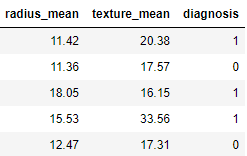Let’s code the KNN:

``````# Defining X and y
X = data.drop('diagnosis',axis=1)
y = data.diagnosis

# Splitting data into train and test
from sklearn.model_selection import train_test_split
X_train, X_test, y_train, y_test = train_test_split(X,y,test_size=0.25,random_state=42)
# Importing and fitting KNN classifier for k=3
from sklearn.neighbors import KNeighborsClassifier
knn = KNeighborsClassifier(n_neighbors=3)
knn.fit(X_train,y_train)
# Predicting results using Test data set
pred = knn.predict(X_test)
from sklearn.metrics import accuracy_score
accuracy_score(pred,y_test)
``````

The above code should give you the following output with a slight variation.

``````0.8601398601398601
``````

What just happened? When we trained the KNN on training data, it took the following steps for each data sample:

1. Calculate the distance between the data sample and every other sample with the help of a method such as Euclidean.
2. Sort these values of distances in ascending order.
3. Choose the top K values from the sorted distances.
4. Assign the class to the sample based on the most frequent class in the above K values.

Let’s visualize how KNN drew a decision boundary on the train data set and how the same boundary is then used to classify the test data set.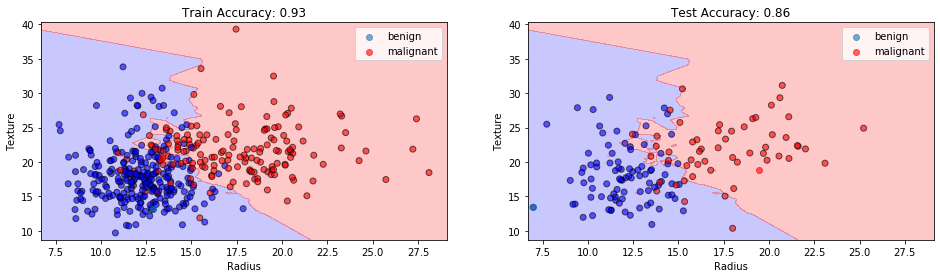KNN Classification at K=3. Image by Sangeet Aggarwal

With the training accuracy of 93% and the test accuracy of 86%, our model might have shown overfitting here. Why so?

When the value of K or the number of neighbors is too low, the model picks only the values that are closest to the data sample, thus forming a very complex decision boundary as shown above. Such a model fails to generalize well on the test data set, thereby showing poor results.

The problem can be solved by tuning the value of _n_neighbors _parameter. As we increase the number of neighbors, the model starts to generalize well, but increasing the value too much would again drop the performance.

Therefore, it’s important to find an optimal value of K, such that the model is able to classify well on the test data set. Let’s observe the train and test accuracies as we increase the number of neighbors.

#knn-algorithm #data-science #knn #nearest-neighbors #machine-learning #algorithms1597235100

## k nearest neighbors computational complexity

### Understanding the computational cost of kNN algorithm, with case study examples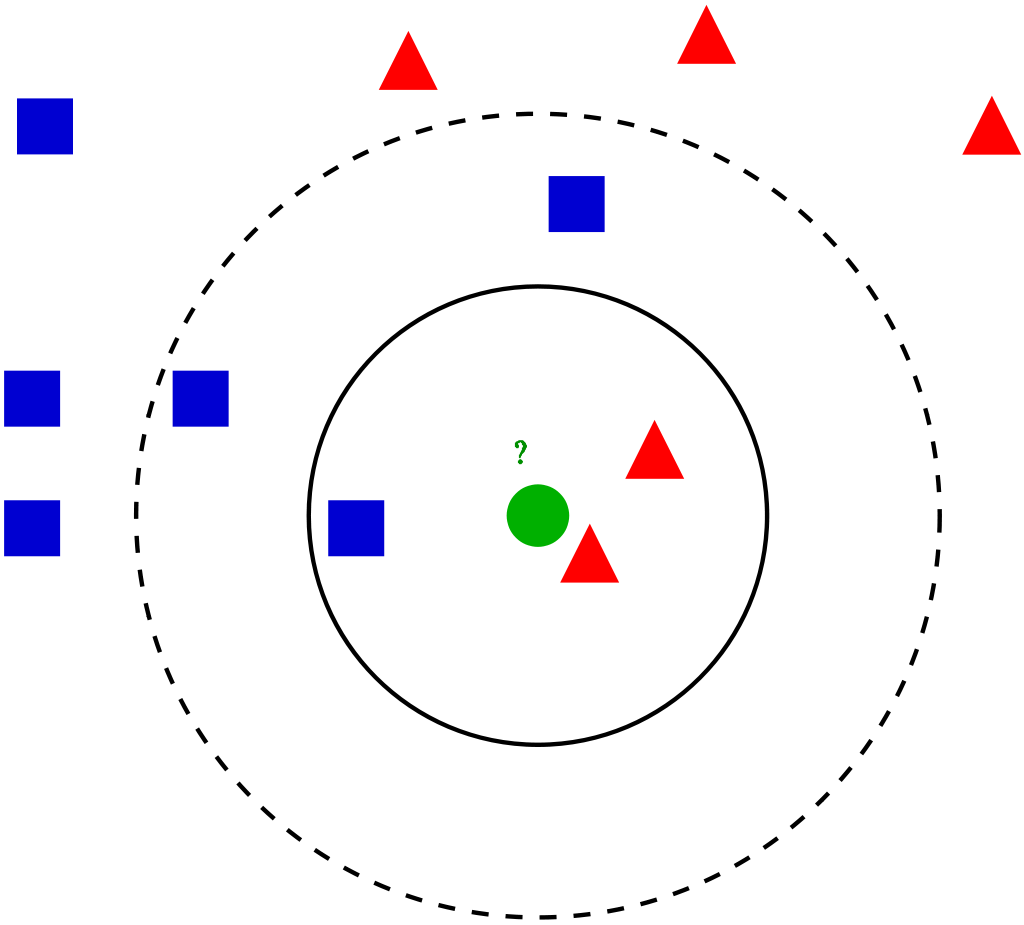Visualization of the kNN algorithm (source)

## Algorithm introduction

kNN (k nearest neighbors) is one of the simplest ML algorithms, often taught as one of the first algorithms during introductory courses. It’s relatively simple but quite powerful, although rarely time is spent on understanding its computational complexity and practical issues. It can be used both for classification and regression with the same complexity, so for simplicity, we’ll consider the kNN classifier.

kNN is an associative algorithm — during prediction it searches for the nearest neighbors and takes their majority vote as the class predicted for the sample. Training phase may or may not exist at all, as in general, we have 2 possibilities:

1. Brute force method — calculate distance from new point to every point in training data matrix X, sort distances and take k nearest, then do a majority vote. There is no need for separate training, so we only consider prediction complexity.
2. Using data structure — organize the training points from X into the auxiliary data structure for faster nearest neighbors lookup. This approach uses additional space and time (for creating data structure during training phase) for faster predictions.

We focus on the methods implemented in Scikit-learn, the most popular ML library for Python. It supports brute force, k-d tree and ball tree data structures. These are relatively simple, efficient and perfectly suited for the kNN algorithm. Construction of these trees stems from computational geometry, not from machine learning, and does not concern us that much, so I’ll cover it in less detail, more on the conceptual level. For more details on that, see links at the end of the article.

In all complexities below times of calculating the distance were omitted since they are in most cases negligible compared to the rest of the algorithm. Additionally, we mark:

• `n`: number of points in the training dataset
• `d`: data dimensionality
• `k`: number of neighbors that we consider for voting

## Brute force method

Training time complexity: `O(1)`

**Training space complexity: **`O(1)`

Prediction time complexity: `O(k * n)`

Prediction space complexity: `O(1)`

Training phase technically does not exist, since all computation is done during prediction, so we have `O(1)` for both time and space.

Prediction phase is, as method name suggest, a simple exhaustive search, which in pseudocode is:

``````Loop through all points k times:
1\. Compute the distance between currently classifier sample and
training points, remember the index of the element with the
smallest distance (ignore previously selected points)
2\. Add the class at found index to the counter
Return the class with the most votes as a prediction
``````

This is a nested loop structure, where the outer loop takes `k` steps and the inner loop takes `n` steps. 3rd point is `O(1)` and 4th is `O(## of classes)`, so they are smaller. Therefore, we have `O(n * k)` time complexity.

As for space complexity, we need a small vector to count the votes for each class. It’s almost always very small and is fixed, so we can treat is as a `O(1)` space complexity.

#k-nearest-neighbours #knn-algorithm #knn #machine-learning #algorithms1596905700

## K-Nearest Neighbors Algorithm

KNN is a non-parametric and lazy learning algorithm. Non-parametric means there is no assumption for underlying data distribution. In other words, the model structure determined from the dataset. This will be very helpful in practice where most of the real-world datasets do not follow mathematical theoretical assumptions.

KNN is one of the most simple and traditional non-parametric techniques to classify samples. Given an input vector, KNN calculates the approximate distances between the vectors and then assign the points which are not yet labeled to the class of its K-nearest neighbors.

The lazy algorithm means it does not need any training data points for model generation. All training data used in the testing phase. This makes training faster and the testing phase slower and costlier. The costly testing phase means time and memory. In the worst case, KNN needs more time to scan all data points, and scanning all data points will require more memory for storing training data.

## K-NN for classification

Classification is a type of supervised learning. It specifies the class to which data elements belong to and is best used when the output has finite and discrete values. It predicts a class for an input variable as well.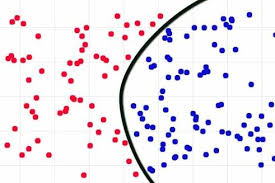Consider given review is positive (or) Negative, classification is all about if we give a new query points determine (or) predict the given review is positive (or) Negative.

Classification is all about learning the function for given points.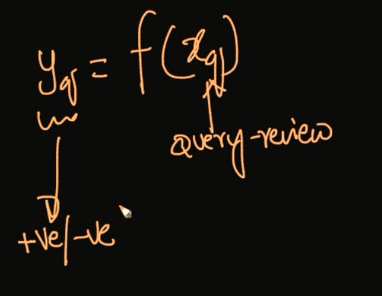How does the K-NN algorithm work?

In K-NN, K is the number of nearest neighbors. The number of neighbors is the core deciding factor. K is generally an odd number if the number of classes is 2. When K=1, then the algorithm is known as the nearest neighbor algorithm. This is the simplest case. Suppose P1 is the point, for which label needs to predict. First, you find the one closest point to P1 and then the label of the nearest point assigned to P1.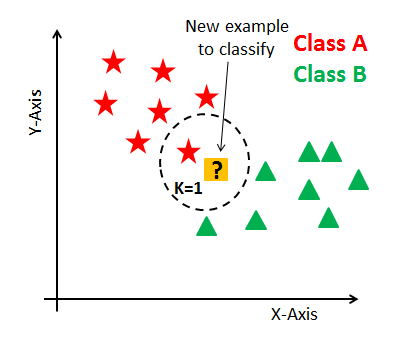Suppose P1 is the point, for which label needs to predict. First, you find the k closest point to P1 and then classify points by majority vote of its k neighbors. Each object votes for their class and the class with the most votes is taken as the prediction. For finding closest similar points, you find the distance between points using distance measures such as Euclidean distance, Hamming distance, Manhattan distance, and Minkowski distance.

K-NN has the following basic steps:

1. Calculate distance
2. Find closest neighbors
3. Vote for labels
4. Take the majority Vote

Failure Cases of K-NN:

_1.When Query Point is far away from the data points.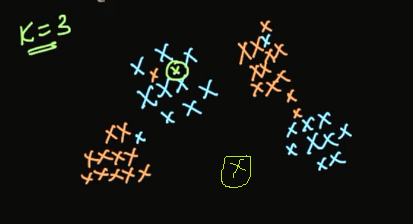2.If we have Jumble data sets.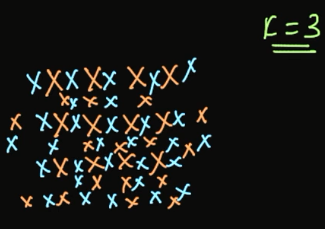For the above image shows jumble sets of data set, no useful information in the above data set. In this situation, the algorithm may be failing.

_Distance Measures in K-NN: _There are mainly four distance measures in Machine Learning Listed below.

1. Euclidean Distance
2. Manhattan Distance
3. Minkowski Distance
4. Hamming Distance

Euclidean Distance

The Euclidean distance between two points in either the plane or 3-dimensional space measures the length of a segment connecting the two points. It is the most obvious way of representing distance between two points. Euclidean distance marks the shortest route of the two points.

The Pythagorean Theorem can be used to calculate the distance between two points, as shown in the figure below. If the points (x1,y1)(x1,y1) and (x2,y2)(x2,y2) are in 2-dimensional space, then the Euclidean distance between them is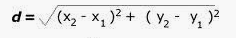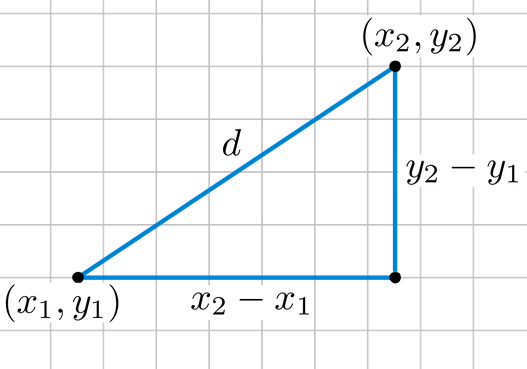Euclidean distance is called an L2 Norm of a vector.

Norm means the distance between two vectors.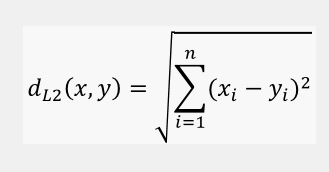Euclidean distance from an origin is given by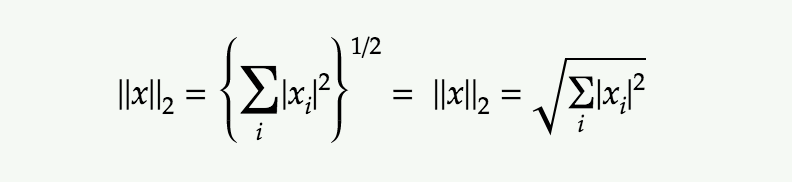Manhattan Distance

The Manhattan distance between two vectors (city blocks) is equal to the one-norm of the distance between the vectors. The distance function (also called a “metric”) involved is also called the “taxi cab” metric.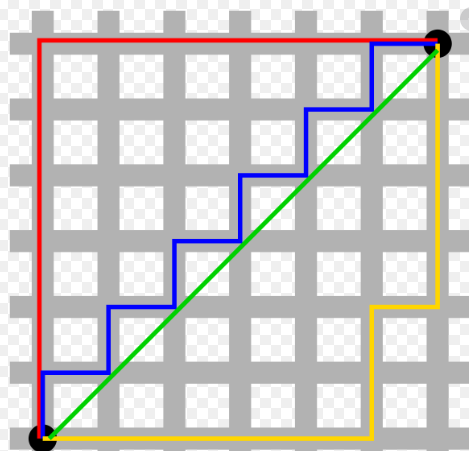Manhattan distance between two vectors is called as L1 Norm of a vector.

In L2 Norm we take the sum of the Squaring of the difference between elements vectors, in L1 Norm we take the sum of the absolute difference between elements vectors.

Manhattan Distance between two points (x1, y1) and (x2, y2) is:

|x1 — x2| + |y1 — y2|.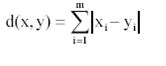Manhattan Distance****from an origin is given by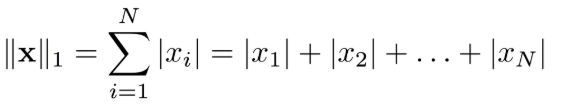Minkowski Distance

Minkowski distance__is a metric in a normed vector space. Minkowski distance is used for distance similarity of vector. Given two or more vectors, find distance similarity of these vectors.

#analytics #machine-learning #applied-ai #data-science #k-nearest-neighbors #data analytic